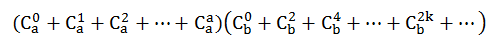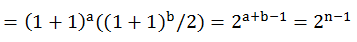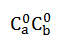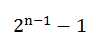# HDU-5363-快速幂-组合数公式

### 分析：

1. 任意个偶数的和是偶数
2. 偶数个奇数的和是偶数
3. 任意个偶数+偶数个奇数的和是偶数### 下面是代码：

#include <iostream>
#include <cmath>

using namespace std;

typedef long long LL ;

const int M = 1000000007;
const int N = 1e5;
int a[N + 1];

LL Pow(int a, int b)
{
LL ans = 1, t = a;
while(b)
{
if (b&1) ans = ans*t%M;
t = t*t%M;
b >>= 1;
}
return ans;
}
int main()
{
LL ans = 0;
int n, a;
int e = 0, o = 0;
cin >> n;
for (int i = 0; i < n; i++) {
cin >> a;
ans = Pow(2, a-1) -1 ;
cout << ans << endl;
}
return 0;
}
••Simple And Clear